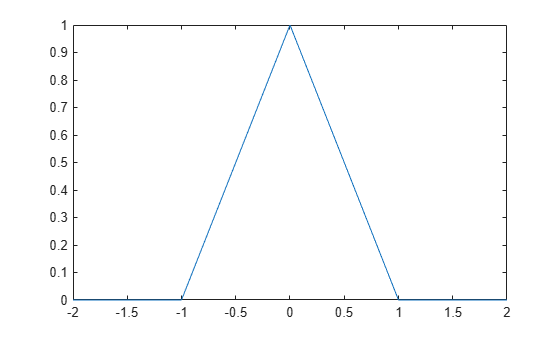# triangularPulse

Triangular pulse function

## Syntax

``triangularPulse(a,b,c,x)``
``triangularPulse(a,c,x)``
``triangularPulse(x)``

## Description

example

````triangularPulse(a,b,c,x)` returns the Triangular Pulse Function.```
````triangularPulse(a,c,x)` is a shortcut for `triangularPulse(a, (a + c)/2, c, x)`.```
````triangularPulse(x)` is a shortcut for `triangularPulse(-1, 0, 1, x)`.```

## Examples

collapse all

```syms x fplot(triangularPulse(x), [-2 2])```Compute the triangular pulse function for these numbers. Because these numbers are not symbolic objects, you get floating-point results.

```[triangularPulse(-2, 0, 2, -3) triangularPulse(-2, 0, 2, -1/2) triangularPulse(-2, 0, 2, 0) triangularPulse(-2, 0, 2, 3/2) triangularPulse(-2, 0, 2, 3)]]```
```ans = 0 0.7500 1.0000 0.2500 0```

Compute the same values symbolically by converting the numbers to symbolic objects.

```[triangularPulse(sym(-2), 0, 2, -3) triangularPulse(-2, 0, 2, sym(-1/2)) triangularPulse(-2, sym(0), 2, 0) triangularPulse(-2, 0, 2, sym(3/2)) triangularPulse(-2, 0, sym(2), 3)]```
```ans = 0 3/4 1 1/4 0```

Use `triangularPulse` with one input argument as a shortcut for computing `triangularPulse(-1, 0, 1, x)`:

```syms x triangularPulse(x)```
```ans = triangularPulse(-1, 0, 1, x)```

Use `triangularPulse` with three input arguments as a shortcut for computing `triangularPulse(a, (a + c)/2, c, x)`:

```syms a c x triangularPulse(a, c, x)```
```ans = triangularPulse(a, a/2 + c/2, c, x)```

Depending on the relation between inputs, the `triangularPulse` has special values.

Compute the triangular pulse function for `a < x < b`:

```syms a b c x assume(a < x < b) triangularPulse(a, b, c, x)```
```ans = (a - x)/(a - b)```

For further computations, remove the assumption by recreating the variables using `syms`:

`syms a b x`

Compute the triangular pulse function for `b < x < c`:

```assume(b < x < c) triangularPulse(a, b, c, x)```
```ans = -(c - x)/(b - c)```

For further computations, remove the assumption:

`syms b c x`

Compute the triangular pulse function for `a = b`:

```syms a b c x assume(b < c) triangularPulse(b, b, c, x)```
```ans = -((c - x)*rectangularPulse(b, c, x))/(b - c)```

Compute the triangular pulse function for `c = b`:

```assume(a < b) triangularPulse(a, b, b, x)```
```ans = ((a - x)*rectangularPulse(a, b, x))/(a - b)```

For further computations, remove all assumptions on `a`, `b`, and `c`:

`syms a b c`

## Input Arguments

collapse all

Input, specified as a number or a symbolic scalar. This argument specifies the rising edge of the triangular pulse function.

Input, specified as a number or a symbolic scalar. This argument specifies the peak of the triangular pulse function. If you specify `a` and `c`, then `(a + c)/2`. Otherwise, `0`.

Input, specified as a number or a symbolic scalar. This argument specifies the falling edge of the triangular pulse function.

Input, specified as a number, vector, matrix, or array, or a symbolic number, variable, array, function, or expression.

collapse all

### Triangular Pulse Function

If `a < x < b`, then the triangular pulse function equals `(x - a)/(b - a)`.

If `b < x < c`, then the triangular pulse function equals `(c - x)/(c - b)`.

If `x <= a` or `x >= c`, then the triangular pulse function equals 0.

The triangular pulse function is also called the triangle function, hat function, tent function, or sawtooth function.

## Tips

• If `a`, `b`, and `c` are variables or expressions with variables, `triangularPulse` assumes that `a <= b <= c`. If `a`, `b`, and `c` are numerical values that do not satisfy this condition, `triangularPulse` throws an error.

• If `a = b = c`, `triangularPulse` returns 0.

• If `a = b` or `b = c`, the triangular function can be expressed in terms of the rectangular function.